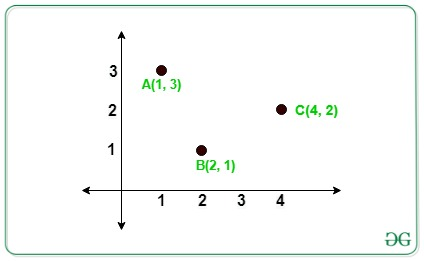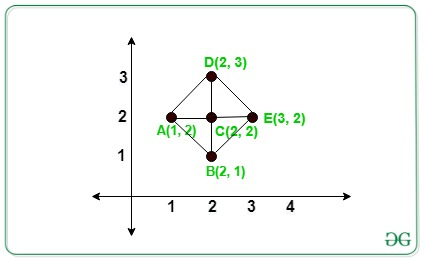GeeksforGeeks App
Open AppBrowser
Continue

# Count of Right-Angled Triangle formed from given N points whose base or perpendicular are parallel to X or Y axis

Given an array arr[] of N distinct integers points on the 2D Plane. The task is to count the number of Right-Angled Triangle from N points such that the base or perpendicular is parallel to the X or Y-axis.

Examples:

Input: arr[][] = {{4, 2}, {2, 1}, {1, 3}}
Output:
Explanation:In the above image there is no right-angled triangle formed.
Input: arr[][] = {{1, 2}, {2, 1}, {2, 2}, {2, 3}, {3, 2}}
Output:
Explanation:In the above image there are 4 right-angled triangles formed by triangles ACB, ACD, DCE, BCE.

Approach: The idea is to store the count of each co-ordinate’s having the same X and Y co-ordinates respectively. Now traverse each given points and the count of a right-angled triangle formed by each coordinate (X, Y) is given by:

Count of right-angled triangles = (frequencies of X coordinates – 1) * (frequencies of Y coordinates – 1)

Below are the steps:

• Create two maps to store the count of points, one for having the same X-coordinate and another for having the same Y-coordinate.
• For each value in the map of x-coordinate and in the map of y-coordinate choose that pair of points as pivot elements and find the frequency of that pivot element.
• For each pivot element(say pivot) in the above step, the count of right-angled is given by:

(m1[pivot].second-1)*(m2[pivot].second-1)

• Similarly, calculate the total possible right-angled triangle for other N points given.
• Finally, sum all the possible triangle obtained that is the final answer.

Below is the implementation of the above approach:

## C++

 `// C++ program for the above approach` `#include ``using` `namespace` `std;` `// Function to find the number of right``// angled triangle that are formed from``// given N points whose perpendicular or``// base is parallel to X or Y axis``int` `RightAngled(``int` `a[], ``int` `n)``{` `    ``// To store the number of points``    ``// has same x or y coordinates``    ``unordered_map<``int``, ``int``> xpoints;``    ``unordered_map<``int``, ``int``> ypoints;` `    ``for` `(``int` `i = 0; i < n; i++) {``        ``xpoints[a[i]]++;``        ``ypoints[a[i]]++;``    ``}` `    ``// Store the total count of triangle``    ``int` `count = 0;` `    ``// Iterate to check for total number``    ``// of possible triangle``    ``for` `(``int` `i = 0; i < n; i++) {` `        ``if` `(xpoints[a[i]] >= 1``            ``&& ypoints[a[i]] >= 1) {` `            ``// Add the count of triangles``            ``// formed``            ``count += (xpoints[a[i]] - 1)``                     ``* (ypoints[a[i]] - 1);``        ``}``    ``}` `    ``// Total possible triangle``    ``return` `count;``}` `// Driver Code``int` `main()``{``    ``int` `N = 5;` `    ``// Given N points``    ``int` `arr[] = { { 1, 2 }, { 2, 1 },``                     ``{ 2, 2 }, { 2, 3 },``                     ``{ 3, 2 } };` `    ``// Function Call``    ``cout << RightAngled(arr, N);` `    ``return` `0;``}`

## Python3

 `# Python3 program for the above approach``from` `collections ``import` `defaultdict` `# Function to find the number of right``# angled triangle that are formed from``# given N points whose perpendicular or``# base is parallel to X or Y axis``def` `RightAngled(a, n):``    ` `    ``# To store the number of points``    ``# has same x or y coordinates``    ``xpoints ``=` `defaultdict(``lambda``:``0``)``    ``ypoints ``=` `defaultdict(``lambda``:``0``)``    ` `    ``for` `i ``in` `range``(n):``        ``xpoints[a[i][``0``]] ``+``=` `1``        ``ypoints[a[i][``1``]] ``+``=` `1``        ` `    ``# Store the total count of triangle``    ``count ``=` `0``    ` `    ``# Iterate to check for total number``    ``# of possible triangle``    ``for` `i ``in` `range``(n):``        ``if` `(xpoints[a[i][``0``]] >``=` `1` `and``            ``ypoints[a[i][``1``]] >``=` `1``):``            ` `            ``# Add the count of triangles``            ``# formed``            ``count ``+``=` `((xpoints[a[i][``0``]] ``-` `1``) ``*``                      ``(ypoints[a[i][``1``]] ``-` `1``))``            ` `    ``# Total possible triangle``    ``return` `count` `# Driver Code``N ``=` `5` `# Given N points``arr ``=` `[ [ ``1``, ``2` `], [ ``2``, ``1` `],``        ``[ ``2``, ``2` `], [ ``2``, ``3` `],``        ``[ ``3``, ``2` `] ]` `# Function call``print``(RightAngled(arr, N))` `# This code is contributed by Stuti Pathak`

## Java

 `// Java program for the above approach``import` `java.util.*;``class` `GFG{` `// Function to find the number of right``// angled triangle that are formed from``// given N points whose perpendicular or``// base is parallel to X or Y axis``static` `int` `RightAngled(``int` `a[][], ``int` `n)``{` `    ``// To store the number of points``    ``// has same x or y coordinates``    ``HashMap xpoints  = ``new` `HashMap();``    ``HashMap ypoints  = ``new` `HashMap();` `    ``for` `(``int` `i = ``0``; i < n; i++)``    ``{``        ``if``(xpoints.containsKey(a[i][``0``]))``        ``{``            ``xpoints.put(a[i][``0``], xpoints.get(a[i][``0``]) + ``1``);``        ``}``        ``else``        ``{``            ``xpoints.put(a[i][``0``], ``1``);``        ``}``        ``if``(ypoints.containsKey(a[i][``1``]))``        ``{``            ``ypoints.put(a[i][``1``], ypoints.get(a[i][``1``]) + ``1``);``        ``}``        ``else``        ``{``            ``ypoints.put(a[i][``1``], ``1``);``        ``}``    ``}` `    ``// Store the total count of triangle``    ``int` `count = ``0``;` `    ``// Iterate to check for total number``    ``// of possible triangle``    ``for` `(``int` `i = ``0``; i < n; i++)``    ``{``        ``if` `(xpoints.get(a[i][``0``]) >= ``1` `&&``            ``ypoints.get(a[i][``1``]) >= ``1``)``        ``{` `            ``// Add the count of triangles``            ``// formed``            ``count += (xpoints.get(a[i][``0``]) - ``1``) *``                     ``(ypoints.get(a[i][``1``]) - ``1``);``        ``}``    ``}` `    ``// Total possible triangle``    ``return` `count;``}` `// Driver Code``public` `static` `void` `main(String[] args)``{``    ``int` `N = ``5``;` `    ``// Given N points``    ``int` `arr[][] = { { ``1``, ``2` `}, { ``2``, ``1` `},``                    ``{ ``2``, ``2` `}, { ``2``, ``3` `},``                    ``{ ``3``, ``2` `} };` `    ``// Function Call``    ``System.out.print(RightAngled(arr, N));``}``}` `// This code is contributed by Rajput-Ji`

## C#

 `// C# program for the above approach``using` `System;``using` `System.Collections.Generic;``class` `GFG{` `    ``// Function to find the number of right``    ``// angled triangle that are formed from``    ``// given N points whose perpendicular or``    ``// base is parallel to X or Y axis``    ``static` `int` `RightAngled(``int``[, ] a, ``int` `n)``    ``{` `        ``// To store the number of points``        ``// has same x or y coordinates``        ``Dictionary<``int``, ``int``> xpoints = ``new` `Dictionary<``int``, ``int``>();``        ``Dictionary<``int``, ``int``> ypoints = ``new` `Dictionary<``int``, ``int``>();` `        ``for` `(``int` `i = 0; i < n; i++)``        ``{``            ``if` `(xpoints.ContainsKey(a[i, 0]))``            ``{``                ``xpoints[a[i, 0]] = xpoints[a[i, 0]] + 1;``            ``}``            ``else``            ``{``                ``xpoints.Add(a[i, 0], 1);``            ``}``            ``if` `(ypoints.ContainsKey(a[i, 1]))``            ``{``                ``ypoints[a[i, 1]] = ypoints[a[i, 1]] + 1;``            ``}``            ``else``            ``{``                ``ypoints.Add(a[i, 1], 1);``            ``}``        ``}` `        ``// Store the total count of triangle``        ``int` `count = 0;` `        ``// Iterate to check for total number``        ``// of possible triangle``        ``for` `(``int` `i = 0; i < n; i++)``        ``{``            ``if` `(xpoints[a[i, 0]] >= 1 &&``                ``ypoints[a[i, 1]] >= 1)``            ``{` `                ``// Add the count of triangles``                ``// formed``                ``count += (xpoints[a[i, 0]] - 1) *``                         ``(ypoints[a[i, 1]] - 1);``            ``}``        ``}` `        ``// Total possible triangle``        ``return` `count;``    ``}` `    ``// Driver Code``    ``public` `static` `void` `Main(String[] args)``    ``{``        ``int` `N = 5;` `        ``// Given N points``        ``int``[, ] arr = {{1, 2}, {2, 1},``                       ``{2, 2}, {2, 3}, {3, 2}};` `        ``// Function Call``        ``Console.Write(RightAngled(arr, N));``    ``}``}` `// This code is contributed by Rajput-Ji`

## Javascript

 ``

Output:

`4`

Time Complexity: O(N+N) i.e O(N)
Auxiliary Space: O(N+N) i.e O(N)

My Personal Notes arrow_drop_up